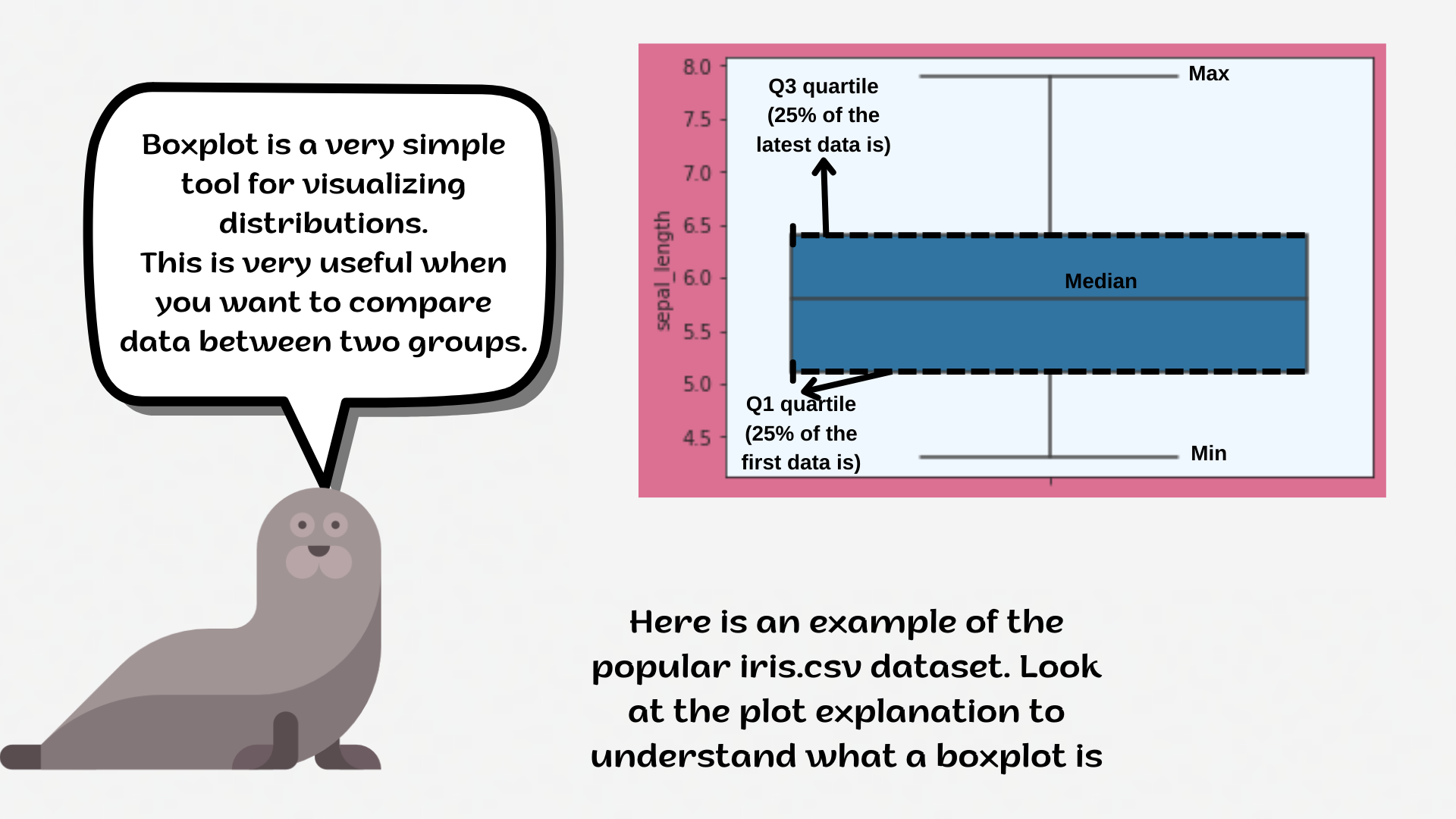Course Content

# Deep Dive into the seaborn Visualization

1. Light Start

2. Distributions of Data

3. Categorical Plot Types

4. Matrix Plots

5. Multi-Plot Grids

6. Regression Models

Deep Dive into the seaborn Visualization

##BoxplotA `boxplot` is a standardized way of displaying the distribution of data based on a five-number summary (minimum, first quartile (Q1), median, third quartile (Q3), and maximum).

It can tell you about your outliers and what their values are. It can also tell you if your data is symmetrical, how tightly your data is grouped, and if and how your data is skewed.1. Set the `'ticks'` style with the `'grey'` `figure.facecolor` and `'white'` `x-` & `yticks`.
2. Create the `boxplot` using the `seaborn` library:
• Set the `x` parameter equals the `'distance'`;
• Set the `y` parameter equals the `'method'`;
• Set the `linewidth` parameter equals `2`;
• Set the `saturation` parameter equals `0.4`;
• Set the `'vlag'` palette.